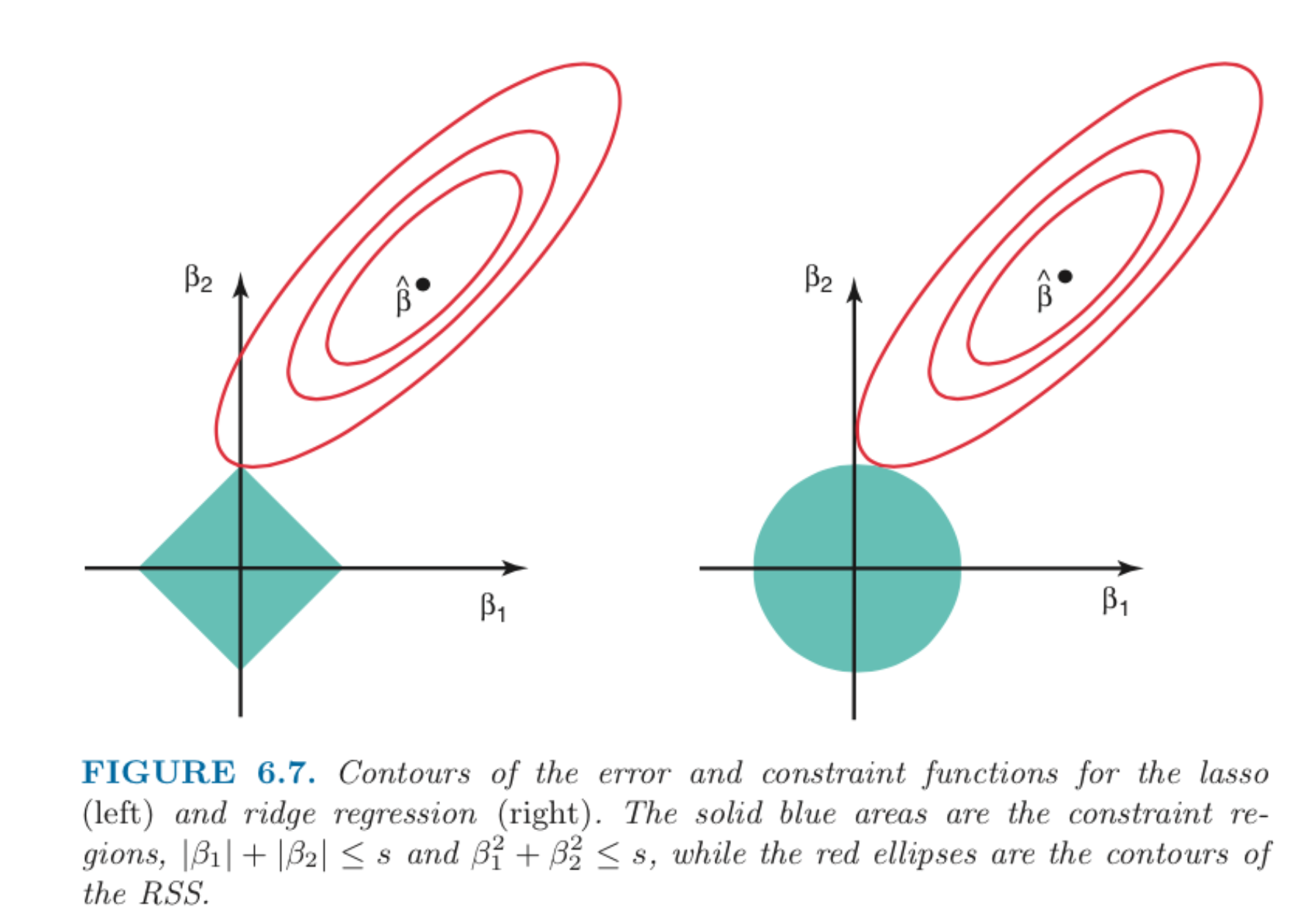# What is Regularization? When do we need it?

Easy Last updated on May 7, 2022, 1:26 a.m.

The real-world data can be noisy and if machine learning capacity is not tuned it will try to capture the noise during training. This attempt of capturing the noise can lead to overfitting, i.e; the model has low training error but very high test/val error. Most of the models require capacity control to avoid overfitting and numerical stability problems in high dimensions. This is accomplished by regularizing the weight parameters during learning. In simpler terms, regularization shrinks the parameters(weights) towards zero, in turn, discouraging learning more complex models.

In Mathematical terms, regularization can be written as:
$$W* = arg min L(f(w, x, y) + \lambda ||w||$$

Here, λ is the tuning parameter that decides how much we want to penalize the flexibility of our model.

In machine learning, there are two types of regularization techniques that are widely used:

1. Ridge Regression: Ridge regression is the name given to regularized least squares when the weights are penalized using the square of the l2 norm.
$$W* = arg min L(f(w, x, y) + \lambda ||w||_{2}^{2}$$

2. Lasso Regression: The Lasso is the name given to regularized least squares when the weights are penalized using the l1 norm. The Lasso problem is a quadratic programming problem. However, it can be solved efficiently for all values of λ using an algorithm called least angle regression (LARS). The advantage of the Lasso is that it simultaneously performs regularization and feature selection.
$$W* = arg min L(f(w, x, y) + \lambda ||w||$$

## Explain how Lasso Regression works as Feature selection?

To understand the working of Lasso and Ridge, we need to understand the working of L2 Norm and L1 Norm. Lets’ assume that we have a model consisting of 2 weight parameters: β1 and β2. say,
$$Y_{pred} = β1 * x_{1} + β2 * x_{2} + β0$$

So, in the case of Ridge Regression, the optimal weight parameters learned will be expressed by β1² + β2² ≤ s, and we know this represents a circle equation as shown in the figure below. This means ridge regression coefficients have the smallest Residual Sum of Squares (RSS) for all points that lie within the circle given by β1² + β2² ≤ s.

On the other hand, the Lasso equation is expressed as |β1|+|β2|≤ s, and we know this represents a closed plane equation as shown in the figure below. Therefore lasso regression coefficients will have minimized error (RSS) for points inside closed diamond plane given by |β1|+|β2|≤ s.Image from: An Introduction to Statistical Learning by Gareth James, Daniela Witten, Trevor Hastie, Robert Tibshirani

The above figure represents equations(green) of lasso(left) and ridge regression(right), along with contour plots for Residual Sum of Squares (RSS)(red). The optimal coefficient estimates of lasso and ridge regression can be seen as the ﬁrst point at which the red contour plot contacts the green constraint equation region.

As ridge regression consists of a circular constraint with no sharp points. The intersection of red and green will not generally happen on an axis, and so the ridge regression coeﬃcient estimates will be exclusively non-zero. Here, axis means (β1=0 or β2=0) so if we recall our model equation from above:
$$Y_{pred} = β1 * x_{1} + β2 * x_{2} + β0$$

If β1!=0 or β2!=0, that means both features have been considered while creating the model. Ridge regression can shrink the coefficients for least important predictors, very close to zero. But it will never make them zero.

Whereas, In the case of Lasso, the constraint equation has corners at each of the axes, making the other axis set to zero. The intersection of red and green will generally happen on an axis. When this occurs, one of the coeﬃcients will turn to zero. In simpler words, the β1 or β2 can be zero, which means both features might not be considered while creating the model.

Similarly, in the case of high dimensional data, many of these coeﬃcients can be zero simultaneously. This helps the lasso method perform feature selection and yield sparse models.

## Can we use the L0.5 Norm or L0 Norm?

As per Wikipedia, the 𝐿0 norm can be used to count the number of nonzero components of a vector, but it lacks homogeneity so can’t be used for optimization. Furthermore, any 𝑙𝑞 norms with 𝑞<1 are not convex in nature, therefore not fit for general ML optimization. The constrain equation in the 𝑙𝑞-norm with 𝑞<1 looks like figure shown below: